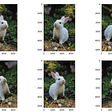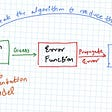# The Missing Library in your Machine Learning Workflow

## A quick guide to using Optuna for hyperparameter optimization

Sound engineers can create the perfect blend in audio by tuning the sliders and knobs to the right positions on audio mixers.

Just like tuning music, machine learning models are also tuned to achieve the best performance.

Before we go into how we can use Optuna for tuning hyperparameters, here’s a quick intro to the topic.

# Hyperparameter Optimization

## What are hyperparameters?

Hyperparameters can be thought of as configuration values that control the learning process of an algorithm.

For example, let’s say you’re building a motorized toy car for a racing competition from scratch. You have control over the car’s specifications, such as the size of the tires, the motor speed, the torque, etc., that will determine how it performs on a race track. If the goal is to win the race, you would configure those settings for optimal performance.

Similarly, many machine learning algorithms also have these settings (hyperparameters) where you can tune or tweak the performance of the models to obtain the best performance.

I say most because simple ones, such as Simple Linear Regression, do not have them.

Below are some examples of model hyperparameters that are configured before model training.

• `k` value in K-Nearest Neighbors
• Learning rate in Neural Networks
• `C` value in Logistic Regression
• `n_components` in Principal Components Analysis
• `max_depth`, `n_estimators`, `max_features`, etc. in Random Forest

## What is hyperparameter optimization?

Now that you understand what hyperparameters are (hopefully), it’s time to understand how to optimize it.

Hyperparameter optimization is the idea of finding the right set of hyperparameters that yields an optimized model which minimizes or maximizes an objective function.

Depending on the metric you want to optimize in a machine learning model, the objective function could return the loss or the accuracy of the model, where the loss is something we want to minimize, and accuracy is something we want to maximize

A function that we want to minimize is called a loss function, which in simple terms, is a way to tell how poorly your machine learning model is performing.

## Why is it important?

Machine Learning models aren’t able to learn the right set of hyperparameters to use by themselves. This is why it’s fundamental to tune them to the right settings so that they can achieve higher predictive power.

## How to do it

There are various algorithms and tools that can be used to perform hyperparameter tuning.

The most common way that many intro courses reach is GridSearchCV, an exhaustive approach, where every possible combination of parameters is used to fit a model and optimize for the best performance. This approach is very expensive and takes a lot of time.

There are other approaches such as RandomizedSearchCV, which randomly samples hyperparameter values to fit the model, and more model-specific approaches such as LogisticRegressionCV and ElasticNetCV.

These approaches have problems and limitations, but there are new packages for hyperparameter tuning that solves these limitations, and one of them is Optuna.

# Introducing Optuna

Optuna is “an automatic hyperparameter optimization software framework, particularly designed for machine learning.

The key features of Optuna are as follows (source)

It’s popular among Kaggle Competitors and well-received by the ML community.

It’s also framework agnostic, which supports any machine learning or deep learning framework.

In this article, we’ll be exploring what Optuna does and try it out with sklearn in a simple example.

As always, here’s where you can find the code for this article:

# Install Dependencies

First, install optuna with pip.

Here, we load the optuna library and sklearn and set the verbosity level to warnings only.

# Basic of Optuna

Let’s first understand three terminologies in Optuna.

• Trial: An execution of the objective function
• Study: An optimization based on the objective function contains a set of trials.
• Parameter: A variable that we want to optimize

We have this quadratic function below, and we want to optimize it.

If you forgot your calculus, optimizing a function means finding an input to the function that results in the minimum or maximum output from the function.

To do that, you first:

1. differentiate the function `2(x-1)`
2. Set the result to zero `2x — 2 = 0`
3. Then you solve for x `x = 1`

Let’s now optimize it with Optuna and see the results.

## Define the objective function

First, we start by defining an objective function.

We can suggest values that we want Optuna to sample from for our hyperparameter in the function.

In our case, `x` is a float number. And we give a range from `-10` to `10` for Optuna to sample from.

In other cases, if our variable was a categorical or an integer, we could use `suggest_categorical`, or `suggest_int` respectively.

Once we have the objective defined, we create the study using `create_study`

Then we call the optimize function on it and set the number of trials.

The `logging_callback` variable passed to the parameter `callbacks` is telling Optuna to only produce an output when the best value is updated and is not required otherwise.

After the study is done optimizing, you can get the results of the best parameter like below.

I coded a custom function below to print out all the useful info of a study, including the best trial of the study.

Here’s what we get when using this function on our study.

## Let’s increase the number of variables for the function.

Now we have a quadratic function with three parameters (x, y, z)

With a bit of calculus or basic math intuition, you can easily figure out the value for x, y, and z that will make this equation equal to zero.

The answer: `x = 1`, `y = 2`, `z = 3`

Let’s use Optuna to optimize this function.

From 100 trials, it seems Optuna can’t find the best value for our variables.

Here comes the best part about Optuna.

It saves the most recent trial, and we can keep optimizing our study until we are satisfied.

Let’s optimize with 500 more trials.

With 600 trials in total, Optuna is able to get closer to the right values.

Now let’s look at Optuna’s built-in functions for visualizing the optimizations.

# Visualizations

## Optimization history

With this function, we can observe at which # of trials does Optuna obtain the best value.

## Objective Values

With `plot_slice`, we can also see as the # trials increase (darker shade), most of the values converge around the right values.

Now let’s try Optuna on a dataset and use it with sklearn to optimize for the right classifier.

# Wine Dataset

We’ll be using the classic wine dataset for this example

We can load the dataset using the sklearn dataset package.

We have a target value we want to classify, which are the different types of wine.

Now our goal is to predict the class and optimize for accuracy.

## Optuna with Sklearn

Below you see an example of integrating Optuna with sklearn.

First we can sample from the classifier algorithms to use — Support Vector Classifier and the Random Forest algorithm

Then, depending on which algorithm was sampled, they have their respective hyperparamers that Optuna can sample.

At the end, the score will be calculated and the accuracy is the value we want to optimize.

Since the higher the accuracy, the better, we create a study where we want to maximize, and we can tell Optuna that like below.

Running 100 trials on the study, we get an accuracy of 96.6% and it tells us the Random Forest Algorithm should be used, with 20 estimators and a maximum depth of 24.

Let’s plot the optimization history.

It seems around the 18 trial mark, the best value was already obtained.

Let’s also plot the hyperparameters as well.

It seems `max_depth` has a lot of variation, and notice how Random Forest has more data points, which maybe suggests it was the better algorithm achieving higher accuracies.

You can also plot hyperparameter importance, which can tell us which hyperparameters are important and which to discard using the `plot_param_importances` function.

This is important because the difficulty of optimization increases roughly exponentially with regard to the number of parameters.

So it’s essential to only optimize for the important parameters.

Check out more visualizations you can do with Optuna.

# Conclusion

This was a short guide to using Optuna. If you want to dive deeper into this tool, check out the resources below.

## Kaggle Notebooks

Want to discuss the latest developments in Data Science and AI with other data scientists? Join our discord server!

Follow the Bitgrit Data Science Publication for more articles like this!

--

--

--

## More from bitgrit Data Science Publication

We’re democratizing AI with our online competition platform — bitgrit.net. On our publication, we publish only high-quality data science-related topics. Become a writer by emailing us at: info@bitgrit.net

## Part 1a: The Convolutional neural network (CNN) intuition guide that even your pet can understand## “Dynamic Selection of Fitness Function in Genetic Algorithm for Feature Selection in Software…## Regression & Classification: Side by side comparison & Concepts.## How To Train Your BERT Model 5X Faster Than In Colab## Image Augmentation to Build a Powerful Image Classification Model## How to understand Machine Learning?## Sematic Segmentation using mmsegmentation## Explaining AlexNet Convolutional Neural Network## Benedict Neo

+1.5M views | Connect 👉 https://linkedin.com/in/benedictneo/

## Machine Learning #1: History and Meaning## Lessons Learned While Scraping Data From Dynamic Sites for my Regression ML Project## A week inside a Data Science Project## What is “Attention”?| From the Basics NLP Part 2/4